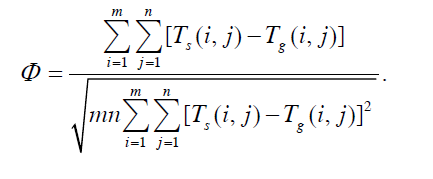# DEFINE_ON_DEMAND 的循环

• 我想使用DEFINE_ON_DEMAND(Phi1)函数计算场协同数然后我的代码

DEFINE_ON_DEMAND(Phi1)
{
face_t f;
Domain *d;
real T_diff,T_diff2,Phi,m,n;
real i = 0.0;
real T_sum = 0.0;
real T_sum2 = 0.0;
cell_t c;
m = 169;	/*m,n 分别表示平面网格个数*/
n = 119;	/*目前节点数为  170x120 */
d = Get_Domain(1);
{
{
begin_c_loop(c,t)
{
T_diff = C_UDSI(c,t,1)-C_UDSI(c,t,0);
T_diff2 = pow(C_UDSI(c,t,1)-C_UDSI(c,t,0),2);
T_sum += T_diff;
T_sum2 += T_diff2;
Message("T_diff is:   %d..\n", T_diff);
Message("T_diff2 is:   %d..\n", T_diff2);
i += 1;
Message("i is:   %d..\n", i);
}
end_c_loop(c,t)
}
Phi = T_sum/pow(m*n*T_sum2,0.5);
Message("count is:   %d..\n", i);
Message("T_sum is:   %d..\n", T_sum);
Message("T_sum2 is:   %d..\n", T_sum2);
Message("Field Synergy Phi is:   %d..\n", Phi);
}
}


C_UDSI(c,t,1),C_UDSI(c,t,0) 分别表示固体和气体温度，不知道哪里有问题？

• DEFINE_ON_DEMAND只在你需要执行的时候执行一次。所以不能再迭代的时候重复使用。如果药循环计算，可以用DEFINE_EXECUTE_AT_END。

• @astremon 对，我就是只是在模拟结束以后计算这个数值，我有看过使用这个函数得到一个自定义内存的场，然后按照我的想法修改了一下，函数里面的循环难道不是挨个获取网格cell的结果吗，还是我的理解有问题，如果可以的话是否可以帮忙把这个公式的代码编写一下（按照我的理解代码应该就是我写的样子，可是我算的结果还是有问题），谢谢！

• @两月三年 你这出来的结果是什么，陷入死循环吗还是？

• A segmentation fault

• 一个单核代码能出错其实还是不常见的